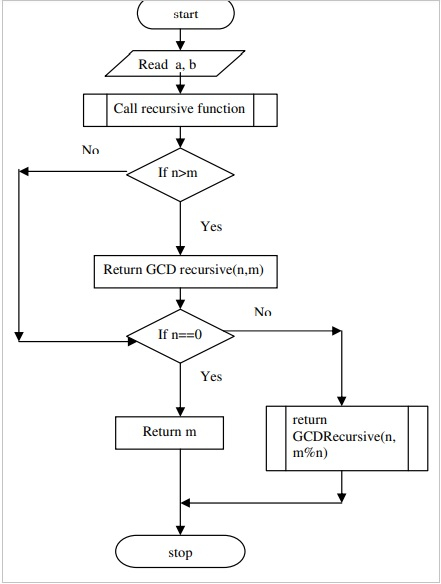# C program to find GCD of numbers using recursive function

## Problem

Find the greatest common divisor (GCD) for the given two numbers by using the recursive function in C programming language.

## Solution

The solution to find the greatest common divisor (GCD) for the given two numbers by using the recursive function is as follows −

## Algorithm

Refer an algorithm given below to find the greatest common divisor (GCD) for the given two numbers by using the recursive function.

Step 1 − Define the recursive function.

Step 2 − Read the two integers a and b.

Step 3 − Call recursive function.

a. if i>j
b. then return the function with parameters i,j
c. if i==0
d. then return j
e. else return the function with parameters i,j%i.

## Flow chart

A flowchart is given below for an algorithm to find the greatest common divisor (GCD) for the given two numbers by using the recursive function.## Example

Following is the C program to find the greatest common divisor (GCD) for the given two numbers by using the recursive function

Live Demo

#include<stdio.h>
#include<math.h>
unsigned int GCD(unsigned i, unsigned j);
int main(){
int a,b;
printf("Enter the two integers: ");
scanf("%d%d",&a,&b);
printf("GCD of %d and %d is %d",a,b,GCD(a,b));
return 0;
}
/* Recursive Function*/
unsigned int GCD(unsigned i, unsigned j){
if(j>i)
return GCD(j,i);
if(j==0)
return i;
else
return GCD(j,i%j);
}

## Output

When the above program is executed, it produces the following result −

Enter the two integers: 4 8
GCD of 4 and 8 is 4